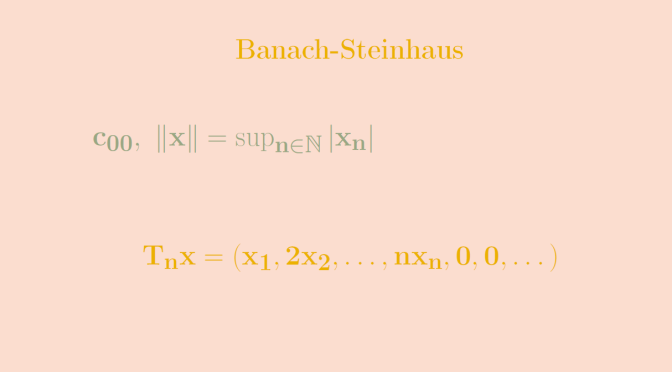# Counterexamples around Banach-Steinhaus theorem

In this article we look at what happens to Banach-Steinhaus theorem when the completness hypothesis is not fulfilled. One form of Banach-Steinhaus theorem is the following one.

Banach-Steinhaus Theorem
Let $$T_n : E \to F$$ be a sequence of continuous linear maps from a Banach space $$E$$ to a normed space $$F$$. If for all $$x \in E$$ the sequence $$T_n x$$ is convergent to $$Tx$$, then $$T$$ is a continuous linear map.

### A sequence of continuous linear maps converging to an unbounded linear map

Let $$c_{00}$$ be the vector space of real sequences $$x=(x_n)$$ eventually vanishing, equipped with the norm $\Vert x \Vert = \sup_{n \in \mathbb N} \vert x_n \vert$ For $$n \in \mathbb N$$, $$T_n : E \to E$$ denotes the linear map defined by $T_n x = (x_1,2 x_2, \dots, n x_n,0,0, \dots).$ $$T_n$$ is continuous as for $$\Vert x \Vert \le 1$$, we have
\begin{align*}
\Vert T_n x \Vert &= \Vert (x_1,2 x_2, \dots, n x_n,0,0, \dots) \Vert\\
& = \sup_{1 \le k \le n} \vert k x_k \vert \le n \Vert x \Vert \le n
\end{align*}

For any $$x \in E$$ there exists $$k \in \mathbb N$$ such that $$x=(x_1, \dots , x_k, 0,0, \dots)$$. Hence for $$n \ge k$$, $$T_n x$$ is constant and equal to $$(x_1,2 x_2, \dots,k x_k,0,0, \dots)$$, which proves that $$(T_n x)$$ converges.

However $$(T_n)$$ is not uniformly bounded as we can see looking at the elements $$\mathbf{1}_n \in E$$ which have all terms equal to $$0$$ except the first $$n$$ ones which are equal to $$1$$:$\Vert \mathbf{1}_n \Vert = 1 \text{ and } \Vert T_n \mathbf{1}_n\Vert = \Vert (1,2, \dots, n, 0,0, \dots) \Vert = n.$ However, this does not contradict Banach-Steinhaus Theorem. $$E$$ is not complete as we can see considering the sequence $$(y_n)$$ of $$E$$ where $$y_n=(1, \frac{1}{2}, \dots, \frac{1}{n},0,0,\dots)$$. $$(y_n)$$ is a Cauchy sequence as for $$1 \le p < q$$: $$\Vert y_q-y_p \Vert=\frac{1}{p+1}$$ but $$(y_n)$$ doesn't converges in $$E$$.

### A discontinuous bilinear map which is separately continuous

A corollary of Banach-Steinhaus Theorem is that if $$E$$ is a Banach space and $$F$$ a normed space, a bilinear map $$B : E \times E \to F$$ which is separately continuous is continuous (as a map of two variables).

We prove here that it might not be the case when $$E$$ is not complete. We take for $$E$$ the vector space of the real polynomials $$\mathbb R[X]$$ equipped with the norm $\Vert P \Vert_1 = \int_0^1 \vert P(t) \vert dt, \ P \in \mathbb R[X]$ and for $$B$$ the bilinear functional defined by $B(P,Q) = \int_0^1 P(t) Q(t) dt, \ P,Q \in \mathbb R[X].$

For $$P$$ fixed we have for any $$Q \in \mathbb R[X]$$
\begin{align*}
\vert B(P,Q) \vert &= \left\vert \int_0^1 P(t) Q(t) dt \right\vert\\
& \le \left(\sup_{t \in [0,1]} \vert P(t) \vert \right) \int_0^1 \vert Q(t) \vert dt\\
&= \left(\sup_{t \in [0,1]} \vert P(t) \vert \right) \Vert Q \Vert_1
\end{align*} which proves that $$B$$ is continuous for the second variable. $$B$$ is also continuous for the first variable as it is symmetric.

Let’s prove that $$B$$ is not continuous. We consider the sequence of polynomials $$(P_n)$$ defined for $$n \in \mathbb N$$ by $$P_n(t)=n^{2/3} t^{n-1}$$. Then $\Vert P_n \Vert_1 = n^{2/3} \int_0^1 t^{n-1} dt = \frac{1}{n^{1/3}} \le 1$ while $\vert B(P_n,P_n) \vert = \int_0^1 P_n^2(t) dt = \frac{n^{4/3}}{2n-1},$ allowing us to conclude as $$\lim\limits_{n \to \infty} \frac{n^{4/3}}{2n-1} = +\infty$$ which proves that $$B$$ is unbounded on the product of two unit balls.

As for the previous example, $$\mathbb R[X]$$ is not complete. This can be proved considering the sequence of polynomials $$(R_n)$$ where $$\displaystyle R_n=\sum_{k=1}^n \frac{t^k}{k}$$. For $$1 \le p < q$$ we have $\Vert R_q-R_p \Vert_1=\int_0^1 \left\vert \sum_{k=p+1}^q \frac{t^k}{k} \right\vert dt \le \frac{1}{p+1}$ Hence $$(R_n)$$ is a Cauchy sequence. However it doesn't converge to a polynomial.